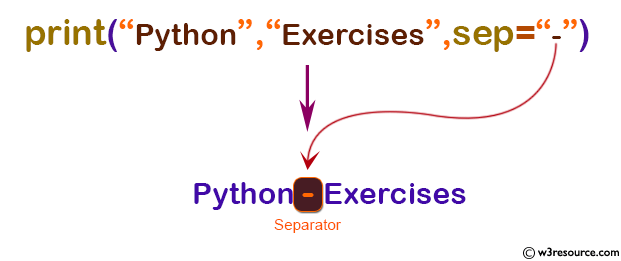# Python: print() function

## print() function

The print() function is used to print objects to the text stream file, separated by sep and followed by end. sep, end and file, if present, must be given as keyword arguments.

Version:

(Python 3.2.5)

Syntax:

```print(*objects, sep=' ', end='\n', file=sys.stdout, flush=False)
```

Parameter:

Name Description Required /
Optional
objects Object to the printed. * indicates that there may be more than one object Optional
sep Objects are separated by sep. Default value: ' ' Optional
end

Specify what to print at the end. Default is line feed ('\n').

Optional
file An object with write(string) method. Default: sys.stdout, which prints objects on the screen. Optional
flush The stream is forcibly flushed If flush is True. Default value: False Optional

Example: Python print() function

``````print("Python Example.")

x = 6
# Two objects are passed
print("x =", x)

y = x
# Three objects are passed
print('x=', x, '= y')

``````

Output:

```Python Example.
x = 6
x= 6 = y
```

Example: Print two messages, using specific separator

``````print("Python Example.")
print("Python", "Exercises", sep="-")
``````

Output:

`Python-Exercises`

Pictorial Presentation:Python Code Editor:

Previous: pow()
Next: property()

Test your Python skills with w3resource's quiz

﻿

## Python: Tips of the Day

How do I check if a list is empty?

For example, if passed the following:

```a = []
if not a:
print("List is empty")
```

Ref: https://bit.ly/2A4JXx9